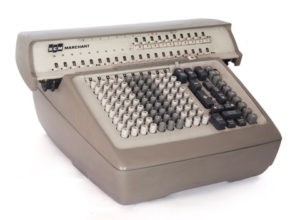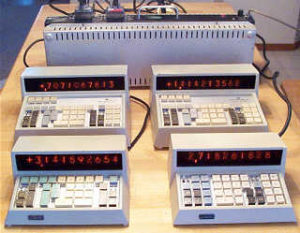# Dividing by Zero

Peter Lobner

In 1967, the old Marchant electromechanical calculators in the physics lab were replaced by silent Wang electronic calculators.

The Marchant SCM Transflo Model TR shown below, manufactured after 1962, was a contemporary of the calculators replaced by the Wang.The Wang 300-series electronic calculators were introduced in October of 1965, and the first machines went into production in March 1966.  The physics lab got a Wang system that looked like this.Source: http://www.oldcalculatormuseum.com/wang360.html

This was the end of an era.  I could no longer vent my frustration at a calculation gone wrong by dividing by zero on several Marchant machines and leaving the room.

Why was this a problem for an electromechanical calculator?  These machines performed multiplication as a series of sequential additions and division were performed as a series of sequential subtractions.  The electromechanical Marchant calculator performed fully automatic division and would grind on until the final result was reached with a balance of zero in the “accumulator”, or until the last register on the machine had been filled and a small positive balance remained in the accumulator.

For example, 16 divided by 3 would be performed as:

16 -3 -3 -3 -3 -3 -3

At this point, the accumulator would be in “overdraft” (a negative value), the last subtraction would be cancelled by adding back +3, yielding a balance of +1 in the accumulator after 5 subtraction cycles. For a result set up for one decimal point accuracy, the calculation would continue as:

1 – 0.3 – 0.3 – 0.3 – 0.3

Again, the accumulator would be in “overdraft”, the last subtraction would be cancelled by adding back + 0.3, yielding a balance of + 0.1 in the accumulator after 3 subtraction cycles.

In this case, the result for 16 divided by 3 would be reported as 5.3.

Division by zero is the sequential subtraction of zero, over and over and over again. It’s an endless loop and the Marchant’s fully automatic division process was happy to oblige until someone got tired of the all the gear noise and cancelled the calculation (or pulled the plug).  These were durable machines.  I came into the lab one morning and found one Marchant still grinding away in an endless loop that probably started late the previous evening (I didn’t do it!).

Watch five old-school calculators grapple with division by zero here: